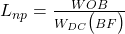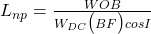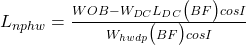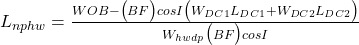Home » Directional Drilling » Neutral Point Calculations In Drilling String

# Neutral Point Calculations In Drilling String

In general, the neutral point can be defined as the point in the drill string where there is a change from tension to compression in the axial stress or the pont that is zero tension and zero compression. The location of this neutral point depends on WOB and the Bf buoyancy factor (that depends on drilling fluid). In practice, the neutral point changes its place as there is a fluctuation in the WOB. For this reason, we call the section that the axial stress keeps changing from tension to compression a “transition zone”.Our objective in this article is to explain to drilling engineers the neutral point definition and calculate the approximate location of the neutral point in a rotary drill string via different formulas.

The neutral point divides the drillstring into two parts. The upper part, with length X 1 , is in effective tension, and the lower part, with length X , is in effective compression.

Fundementals of drilling engineering book

Drilling string components in the “transition zone” may, therefore, alternately experience compression and tension. These cyclic changes can hurt these tools. An excellent example of that is the drilling jars, whose life may be dramatically trimmed if the jars are found in the transition zone. It is also essential, as previously explained, to know if any drill pipe is being run in compression. Thus it is critical to know the location of the neutral point and redesign your BHA to move any critical components out from the transition zone.

To reduce the drill collar buckling tendency, it is aimed to do drill string design in such a way that the neutral point is located inside D/Cs. This design criterion is often used to evaluate the length of required collars.

Drilling Engineering Dipl.-Ing. Wolfgang F. Prassl Curtin University of Technology

## Calculation of Neutral Point In Drilling

Neutral Point calculations formulas in drill string have four cases depending on the well profile and the position of a neutral point that are as follows:

## Neutral Point Formulas In Drilling String

### The Drill Collars In Vertical WellsWhere:

• Lnp: the length from the drilling bit to the neutral point.
• BF: the buoyancy factor
• WDC: the weight per foot of the drill collars

### For HWDP In Vertical Wells

Here, we assume that there are D/Cs & HWDP in the bottom hole assembly. in this situation, It may be more difficult to perform the neutral point calculations. But it will be easier to do the following steps:

1. Check from the previous neutral point formula if it is in the drill collar
2. If Lnp is less than the length of the D/Cs, then the neutral point position is within the D/Cs
3. If Lnp is more than the length of the D/Cs, use the following formula to locate it in HWDPWhere:

• Lnphw: the length from the bottom of HWDP t to the neutral point
• WOB: the weight on bit
• WDC: the weight per foot of the collars
• LDC: The total length of the drill collars
• Whwdp: the weight per foot of the heavy weight
• BF: the Buoyancy Factor

### For The Drill Collars In Deviated Wells

When the neutral point is in the D/Cs section assuming that they have a similar diameter, the following formula shall be used:Where:

• Lnp: the length from drilling bit to neutral point in feet
• WDC: the weight per foot of the drill collars
• BF: the Buoyancy Factor
• WOB: the weight on bit
• I: the wellbore inclination angle

### For HWDP In Vertical Wells

Similar steps are to be performed to check if the neutral point in the D/Cs or the HWDP. If the neutral point is confirmed to be in the HWDP and all the drill collars are of the same diameter, the following formula should be used:Where:

• Lnphw: the length from the bottom of the Heavy weight drill pipe to the neutral point.
• LDC: the total length of the drill collar section.
• Whwdp: the weight per foot of the HWDP

## General Drilling Formula for Neutral Point in Deviated Wells

The last formula can be expanded in the case of a “tapered” bottom hole assembly with drill collars of more than one diameter. For example, if there were two drill collar sizes and the neutral point located in the HWDP, the formula would become:Where:

• WDC1: the weight per foot of the 1st size of drill collar
• LDC1: is the weight per foot of the 1st size of drill collar
• WDC2: the weight per foot of the 2nd size of drill collar
• LDC2: is the weight per foot of the 2nd size of drill collar